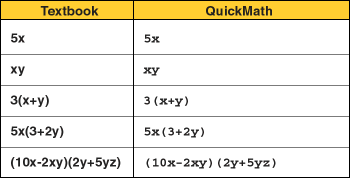Algebra

Equations

Inequalities

Graphs

Numbers

Calculus

Matrices

Tutorials

### Help: Examples of multiplication

Here are some examples of typical expressions involving multiplication. This table shows you what they would look like in a textbook and how they would need to be entered for QuickMath.# Multiplication of Whole Numbers

The product of two whole numbers a and b is defined to be the whole number a * b, which is another name for the sum

b+b+b+ ..... +b         a terms of b

The a and b are called factors of the product.

EXAMPLE   3·4 = 4+4+4      3 terms of 4

MULTIPLICATION BY ZERO

For any number a E W,

a*0 = 0 + 0 + 0   a terms of 0, thus a*0 = 0

EXAMPLE 6*0 = 0

The product of two specific numbers such as 5 and 3 is denoted by

5 *3, 5 x 3, 5(3) or (5)(3)

The product of a specific number and a literal number such as 3 and a is denoted by

3*a, 3 x a,  3(a),  (3)(a) or simply 3a

When we multiply a specific number and a literal number, we write the specific number as the first factor.

The product of two literal numbers such as a and b is denoted by

a*b, a x b a(b), (a)(b), or simply ab

The commutative law of multiplication

For any two numbers a, b E W,

The following are laws of multiplication of whole numbers:

ab = ba

The associative law of multiplication

For any three numbers a. b, c E W,

a(bc) = (ab)c

Example: 5 x (8 x 7) = (5 x 8) x 7

The identity for multiplication

There is a unique number l, called the multiplicative identity, such that for any number a E W,

a x l = 1 x a = a

Example 9 x l = l x 9 = 9

The distributive law of multiplication over addition

For any three numbers a, b, c E W,

(b + c)a = a(b + c) == ab + ac

Example:  4(a + b) = 4a + 4b

Note Although multiplication is a binary operation, it can be extended to find the product c three or more numbers as was done for addition.

Example: 6 x 5 x 3 = (6 x 5) x 3 = 30 x 3 = 90

Or 6 x 5 x 3 = 6 x (5 x 3) = 6 x 15 = 90

Note When an expression involves additions and multiplications without grouping symbols. we perform multiplications before additions.

Example 1. 7 x 8 + 2 = 56 + 2 = 58

Note When an expression involves grouping symbols with only specific numbers inside them it is easier to perform the operations inside the grouping symbols first.

Example  7(3 + 8) + 9 = 7(11) + 9 = 77 + 9 = 86